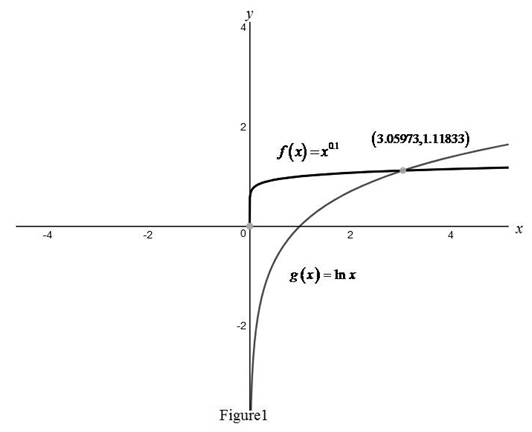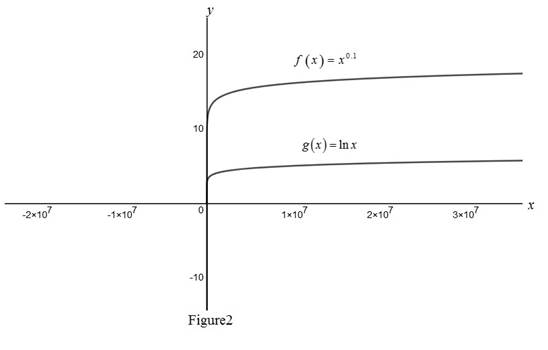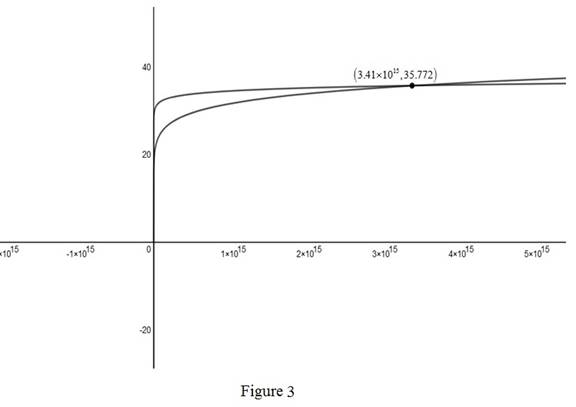# To compare: The graphs of f ( x ) = x 0.1 and g ( x ) = ln x and find the point where graph of g surpasses graph of f .### Single Variable Calculus: Concepts...

4th Edition
James Stewart
Publisher: Cengage Learning
ISBN: 9781337687805### Single Variable Calculus: Concepts...

4th Edition
James Stewart
Publisher: Cengage Learning
ISBN: 9781337687805

#### Solutions

Chapter 1.6, Problem 46E
To determine

## To compare: The graphs of f(x)=x0.1 and g(x)=lnx and find the point where graph of g surpasses graph of f.

Expert Solution

The curve of f(x) surpasses the curve of g(x) at the point x=3.431×1015 .

### Explanation of Solution

Graphs:

Use online graphing calculator and draw the graph of the functions f(x)=x0.1 and g(x)=lnx as shown in the Figure1,From Figure1, observe that f(x) and g(x) has only one intersection point (3.05973,1.11833) .and if 0<x<3.05973 , then f(x)g(x) .

Zoom in the graph in Figure 1 and draw the graph of the given function as shown in below Figure 2,From Figure2, observe that the curve of f(x) approaches g(x) when x is large.

By zooming Figure2, draw the graph of the function as shown in below Figure 3,From Figure3, observe that f(x) is equal to g(x) at point (3.431×1015,35.772) .

Therefore, the curve f(x) surpasses the curve g(x) is at the point x=3.431×1015 .

### Have a homework question?

Subscribe to bartleby learn! Ask subject matter experts 30 homework questions each month. Plus, you’ll have access to millions of step-by-step textbook answers!